﻿ 高速动车组列车轴箱轴承振动监测与故障分析 Vibration Monitoring and Failure Analysis of the Axle Box Bearings for High-Speed EMU

Dynamical Systems and Control
Vol. 09  No. 01 ( 2020 ), Article ID: 33721 , 10 pages
10.12677/DSC.2020.91004

Vibration Monitoring and Failure Analysis of the Axle Box Bearings for High-Speed EMU

Hui Li1,Weixing Cai1, Ning Han1, Li Ma2

1CRRC Qingdao Sifang Co., Ltd., Qingdao Shandong

2HUST‐Wuxi Research Institute, Wuxi JiangsuReceived: Dec. 11th, 2019; accepted: Dec. 24th, 2019; published: Dec. 31st, 2019ABSTRACT

The axle-box bearings are the key components of high-speed EMU train, whose performance and fault directly affect the operating safety. Therefore, it is of great significance to monitor the motion state of bearings and to accurately analyze and predict their faults. In order to monitor the vibration and analyze fault of axle-box bearing, the software and hardware systems of vibration monitoring are designed and used to test vibration experiments. Then, the fault bearing can be effectively diagnosed by time-frequency domain analysis based on the collected data. The vibration monitoring systems of axle-box bearing can provide technical support and ensure safety of the high-speed EMU trains.

Keywords:High-Speed EMU, Bearing Vibration, Testing System, Envelope Spectrum, Fault Analysis1中车青岛四方机车车辆股份有限公司，山东 青岛

2华中科技大学无锡研究院，江苏 无锡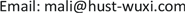1. 引言

2. 高速动车组列车轴箱轴承振动系统搭建

2.1. 硬件系统搭建

2.2. 软件系统设计Figure 1. The data collect software of the axle box bearings for high-speed EMU

2.3. 包络谱

$H\left[{c}_{i}\left(t\right)\right]=\frac{1}{\pi }{\int }_{-\infty }^{+\infty }\frac{{c}_{i}\left(\tau \right)}{t-\tau }\text{d}\tau$ (1)

$z\left(t\right)={c}_{i}\left(t\right)+jH\left[{c}_{i}\left(t\right)\right]$ (2)

$B\left(t\right)=\sqrt{{c}_{i}^{2}\left(t\right)+{H}^{2}\left[{c}_{i}\left(t\right)\right]}$ (3)

3. 高速动车组列车试验台及传感器布置

3.1. 高速动车组列车试验台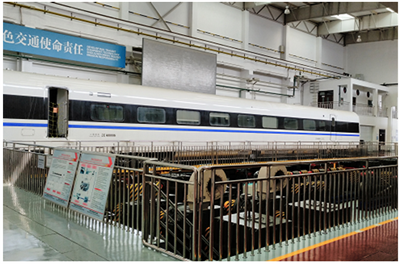Figure 2. The test-bed of high-speed EMU

3.2. 振动传感器布置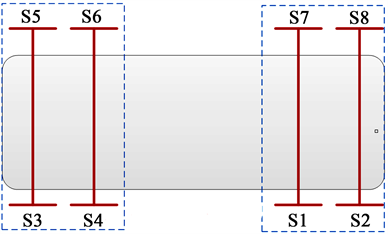(a) 传感器布置图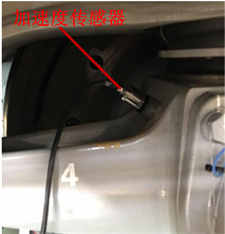(b) 传感器布置实物图

Figure 3. The sensors’ layout of the axle box bearings for high-speed EMU

3.3. 轴箱轴承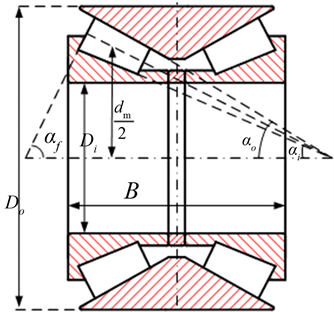Figure 4. The structure of double row tapered roller bearingTable 2. The structure parameters of the axle box bearings for high-speed EMU

${f}_{0}=Z{f}_{r}\left(1-d\mathrm{cos}\alpha /{d}_{m}\right)/2$ (4)

${f}_{i}=Z{f}_{r}\left(1+d\mathrm{cos}\alpha /{d}_{m}\right)/2$ (5)

${f}_{b}=D{f}_{r}\left(1-{\left(d/{d}_{m}\right)}^{2}{\mathrm{cos}}^{2}\alpha \right)/2d$ (6)

${f}_{c}={f}_{r}\left(1-d/{d}_{m}\mathrm{cos}\alpha \right)/2$ (7)

3.4. 试验工况Table 3. The Characteristic frequency of CRI-2692 bearing failure

4. 高速动车组列车轴箱轴承故障诊断

4.1. 正常轴承振动分析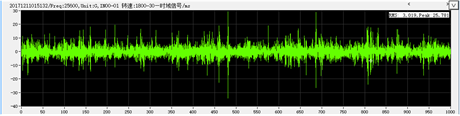(a) 时域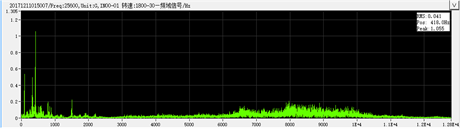(b) FFT(c) 包络谱

Figure 5. Vibration test signal of normal bearing S1 test point (100 km/h)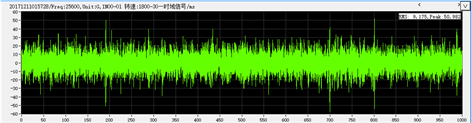(a) 时域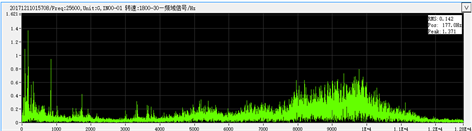(b) FFT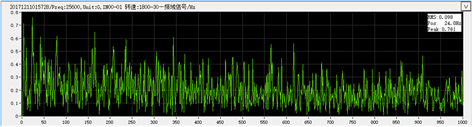(c) 包络谱

Figure 6. Vibration test signal of normal bearing S1 test point (200 km/h)

(a) 时域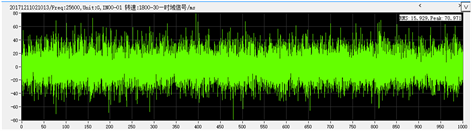(a) 时域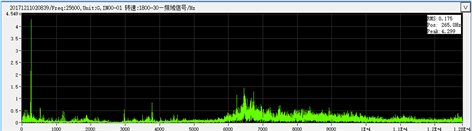(b) FFT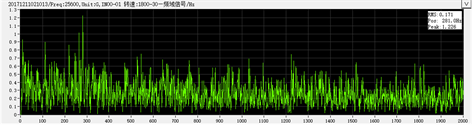(c) 包络谱

Figure 7. Vibration test signal of normal bearing S1 test point (300 km/h)

4.2. 故障轴承振动分析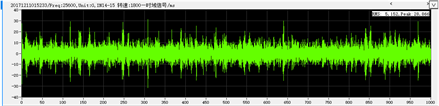(a) 时域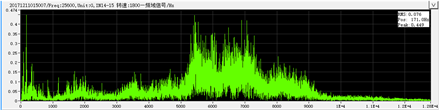(b) FFT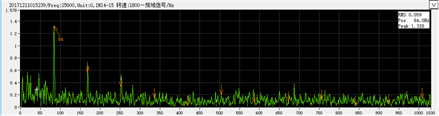(c) 包络谱

Figure 8. Vibration test signal of failed bearing S8 test point (100 km/h)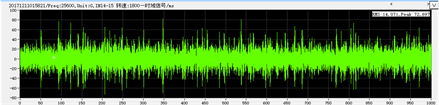(a) 时域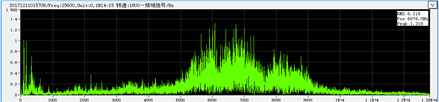(b) FFT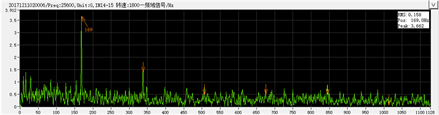(c) 包络谱

Figure 9. Vibration test signal of failed bearing S8 test point (200 km/h)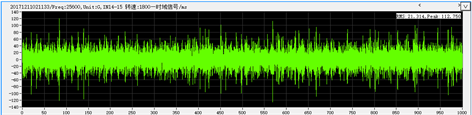(a) 时域(b) FFT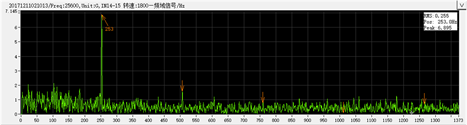(c) 包络谱

Figure 10. Vibration test signal of failed bearing S8 test point (300 km/h)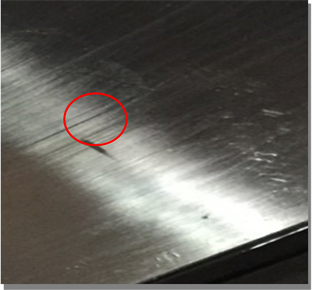Figure 11. The picture of failed bearing

5. 结论

1) 本文设计搭建的高速动车组列车轴箱轴承振动监测系统可以有效测试轴箱轴承的振动信号；

2) 采用包络谱等频域算法分析高速动车组列车轴箱轴承振动，并轴承实物进行对比表明，有效诊断出故障轴承。

《高速动车组关键零部件智能制造新模式应用》项目。

Vibration Monitoring and Failure Analysis of the Axle Box Bearings for High-Speed EMU[J]. 动力系统与控制, 2020, 09(01): 40-49. https://doi.org/10.12677/DSC.2020.91004

1. 1. Li, C., Oliveira, de Oliveira, J.V., Cerrada, M., et al. (2016) Observer-Biased Bearing Condition Monitoring: From Fault Detection to Multi-Fault Classification. Engineering Applications of Artificial Intelligence, 50, 287-301.
https://doi.org/10.1016/j.engappai.2016.01.038

2. 2. 林京, 屈梁生. 基于连续小波变换的信号检测技术与故障诊断[J]. 机械工程学报, 2000, 36(12): 95-100.

3. 3. 吴春光, 房晓华, 化麒. 基于零空间追踪算法的滚动轴承故障诊断[J]. 航空维修与工程, 2016(10): 79-82.

4. 4. 陶洁, 刘义伦, 杨大炼, 宾光富. 基于细菌觅食决策和深度置信网络的滚动轴承故障诊断[J]. 振动与冲击, 2017, 36(23): 68-74.

5. 5. 张琛, 赵荣珍, 邓林峰. 基于变分模态分解奇异值熵的滚动轴承微弱故障辨识方法[J]. 振动与冲击, 2018, 37(21): 87-91+107.

6. 6. 江志农, 胡明辉, 冯坤, 贺雅. 阶次跟踪能量算子与奇异值分解结合的滚动轴承故障诊断[J]. 轴承, 2018(11): 52-56.

7. 7. 尚万峰, 赵升吨, 韩捷. 基于高阶累积量自适应算法的列车轴承的故障诊断[J]. 振动工程学报, 2006, 19(2): 234-237.

8. 8. 刘方, 沈长青, 何清波, 等. 基于时域多普勒校正和EEMD的列车轴承道旁声音监测故障诊断方法研究[J]. 振动与冲击, 2013, 32(24): 104-109.

9. 9. 王靖, 陈特放, 黄采伦, 等. 列车轴承频带变化类故障的诊断方法[J]. 北京工业大学学报, 2012(5): 678-682.

10. 10. Lei, Y., Lin, J., He, Z., et al. (2011) Application of an Improved Kurtogram Method for Fault Diagnosis of Rolling Element Bearings. Mechanical Systems and Signal Processing, 25, 1738-1749.
https://doi.org/10.1016/j.ymssp.2010.12.011

11. 11. 张涛. 机车轴承故障诊断系统的研究与设计[D]: [硕士学位论文]. 长沙: 中南大学, 2005.